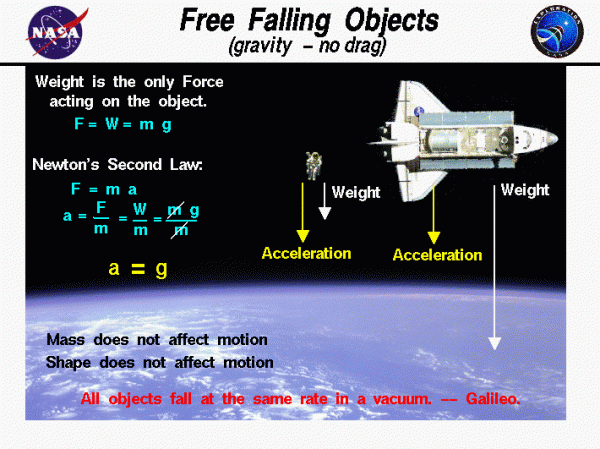# Free Falling Objects## Falling through Vacuum

An object that falls through a vacuum is subjected to only one external force, the gravitational force, expressed as the weight of the object. The weight equation defines the weight W to be equal to the mass of the object m times the gravitational acceleration g:

$$\LARGE W=mg$$

## Gravitational Acceleration

The value of g is 9.8 meters per square second (32.2 feet per square second) on the surface of the Earth and has different values on the surface of the Moon and Mars. The gravitational acceleration g decreases with the square of the distance from the center of the planet. But for many practical problems, we can assume this factor to be a constant. The mass of an object does not depend on the location, the weight does.

## Free Falling

An object that moves because of the action of gravity alone is said to be free falling. If the object falls through an atmosphere, there is an additional drag force acting on the object and the physics involved with the motion of the object is more complex than in free fall. For an object in free fall, we can easily predict the motion of the object.

## Newton’s Second Law of Motion

Assuming the mass of the object remains constant, and the size and speed of the object is not so small or so fast that we must consider relativistic effects, the motion of the object is described by Newton’s second law of motion, force F equals mass m times acceleration a:

$$\LARGE F=ma$$

We can do a little algebra and solve for the acceleration of the object in terms of the net external force and the mass of the object:

$$\LARGE a=\frac{F}{m}$$

For a free-falling object, the net external force is just the weight of the object:

$$\LARGE F=W$$

Substituting into the second law equation gives:

$$\LARGE a=\frac{W}{m}=\frac{mg}{m}=g$$

## Acceleration

The acceleration of the object equals the gravitational acceleration. The mass, size, and shape of the object are not a factor in describing the motion of the object. So, all objects, regardless of size or shape or weight, free fall with the same acceleration.

On the figure, we show an orbiting Space Shuttle and a space walking astronaut. The astronaut and the Shuttle have very different weight, size and shape. But objects in orbit are in a free fall and the only force acting on the objects is the gravitational attraction of the Earth. So, both the astronaut and the Shuttle are accelerated towards the Earth with the same acceleration. Because the objects orbit at some altitude above the Earth’s surface, the acceleration is slightly less than the surface value. At a 200 mile orbit the acceleration is about 90% of the surface value. Since both Space Shuttle and astronaut are falling with the same acceleration, the astronaut appears to be “weightless” and “floats” relative to the Shuttle.

## Ballistic Flight Equations

If you know the local value of the gravitational acceleration, you can use the equations for translation of an object to obtain the instantaneous velocity and location as a function of time. The mass must remain constant for a constant acceleration to occur. If one launches an object from the surface of a planet, and there is only gravity acting on the object (no thrust and no drag), the resulting trajectory is described by the ballistic flight equations.

The remarkable observation that all free-falling objects fall with the same acceleration was first proposed by Galileo, nearly 400 years ago. Galileo conducted experiments using a ball on an inclined plane to determine the relationship between the time and distance traveled. He found that the distance depended on the square of the time and that the velocity increased as the ball moved down the incline. The relationship was the same regardless of the mass of the ball used in the experiment. The story that Galileo demonstrated his findings by dropping two cannon balls off the Leaning Tower of Pisa is just a legend.

Provide feedback